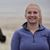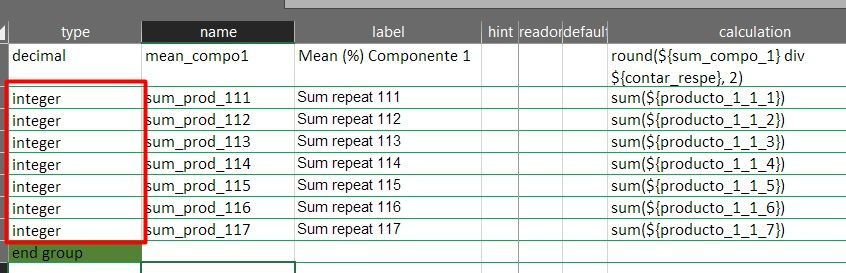# Sum values from multiple repeat fields

2576
6
11-05-2019 09:36 AM
Status: OpenOccasional Contributor III

Currently, it is possible to sum the values across one repeat using the syntax sum(\${survey_question}) within your XLS form.

I would like to see the ability to create an equation that can deal with multiple sum statements, allowing survey designers the ability to add/subtract the total value summed across one repeat field with the total value summed across another repeat field.

For example:

sum(\${survey_question1}) + sum(\${survey_question2})

sum(\${survey_question1}) - sum(\${survey_question2})

sum(\${survey_question1}) + sum(\${survey_question2}) - sum(\${survey_question3})

Esri Technical Support stated "since there is already a workflow to reach our end goal (able to sum one field) we don't think the enhancement will go far." I'm hoping that others out there would also like this ability so this gets some traction with the Survey123 development team.I have the same issue, not sure if this is working. Is working for me, to sum repeated question.

However, I have an unresolved issue, I have 7 repeated integer questions, that I need to sum in order to estimate the mean.  I used the count  function so I can then estimate the mean = total sum / total count answer question.

The problem is that not always the 7 repeated questions will have a response, so that value will be NA or 0. I try to use coalesce to solve this issue, but is not working.

I don't want the 0 value counted in the count function towards estimating the mean.

I have try to get around this issue.

Example:

coalesce(sum(\${producto_1.1.1})+sum(\${producto_1.1.2})+sum(\${producto_1.1.3}),0)....

or

sum(coalesce(\${producto_1.1.1},0))+sum(coalesce(\${producto_1.1.2},0)).....

Maybe @DougBrowning or @IsmaelChivite

have an idea?

Cheers,

FedericobyI think you want

coalesce(sum(\${producto_1.1.1}),0)+coalesce(sum(\${producto_1.1.2}),0).....

I am also not sure Arc will let you have a . in the field name since it uses that in joins.  Not sure.

Hope that helpsthanks for you response.

I try this, but still have issues with the formatting and the sum of the repeats.

When I choose "hidden" or "calculate" in the type column the calculation do not work.

I can't upload my XLSForm here for some reason.

Cheers,

FedericobyWhat type is producto_1_1_1?   I think seeing the form would help but I do not see an attachment option in the editor either.

There must be a way to attach right?  Weird  Maybe the 123 team knows how.Hi ,

I have created a new post with the XSLForm attached

Cheers,

FedericoI am way late to respond here, but in case you are still having issues, this is how I've dealt with a similar situation to what you're describing.

Within a repeat I have 3 questions that could all be answered, or only one or two could be answered. Each of the 3 questions could write a value to one of ~93 unique fields depending on a previous selection. Then, to get a sum I use a series of coalesce statements within my repeat. Outside of my repeat, I then use a sum on the coalesced values. This works well and I would imagine it would also work for your mean calculation. I've included an example below.

Inside Repeat (integer type):

Field 1 = Violation1aFirst (called w/ relevant statement)

Field 2 = Violation1aSecond (called w/ relevant statement)

Field 3 = Violation1aThird (called w/ relevant statement)

Inside Repeat, to get valid value (calculate type):

Field = CoalesceVio1a

coalesce(\${Violation1aFirst},0) + coalesce(\${Violation1aSecond},0) + coalesce(\${Violation1aThird},0)

Outside Repeat to get sum across repeats (integer type):

sum(\${CoalesceVio1a})

This process results in a lot of fields within my survey (all hidden based on relevance or because "calculate" type). All total, I have 526 rows in my XLS form dedicated to dealing with these calculations.